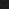JavaPerspective.com  >   Beginner Tutorials  >   3. Java Basics  >   3.9. The if-else statement

3.9. The if-else statement
Last updated: 23 January 2013.

The if-else statement can be used in 3 different ways:

3.9.1. The if statement

An if statement looks something like this:

if(condition){

// statements to be executed only if "condition" is true
}

condition is either a boolean or an expression that evaluates to a boolean. In the example below, the statements c1 = 'a'; and c2 = 'v'; will be executed only if the expression x <= 0 evaluates to true.

Create a class named IfElseStatement and write the code below. The class App shown after the class IfElseStatement calls the method ifElseStatementTest.

public class IfElseStatement{

public void ifElseStatementTest(){

int x = 0;

char c1 = 'z';

char c2 = 'z';

if(x <= 0){

c1 = 'a';
c2 =
'v';

}

System.out.println(c1);
System.out.println
(c2);

}
}

public class App {

public static void main(String[] args) {

new IfElseStatement().ifElseStatementTest();

}

}

The output is:

a
v

If there is only one statement to be executed when the condition is true, you can omit the opening and closing braces as in the example below:

public class IfElseStatement{

public void ifElseStatementTest(){

int x = 0;

char c1 = 'z';

if(x <= 0)

c1 = 'a';

System.out.println
(c1);

}
}

3.9.2. The if-else statement

In the previous section (3.9.1), when the condition is false, the statements contained in the if-block are not executed and the JVM simply proceeds with the statements following the if block, if any. If you explicitly want some statements to be executed when the condition is false, you must add an else-block by using the keyword else this way:

public class IfElseStatement{

public void ifElseStatementTest(){

int x = 0;

char c1 = 'z';

char c2 = 'z';

if(x <= 0)

c1 = 'a';

else

c2 = 'a';

System.out.println
(c1);
System.out.println
(c2);

}
}

The output is:

a
z

If there are multiple statements in the if-block, you must enclose them in opening and closing braces. Likewise, if there are multiple statements in the else-block, you must enclose them in opening and closing braces as shown in the example below:

public class IfElseStatement{

public void ifElseStatementTest(){

int x = 0;

char c1 = 'z';

char c2 = 'z';

if(x <= 0){

c1 = 'a';
c2 =
'a';

}

else{

c1 = 'v';
c2 =
'v';

}

System.out.println(c1);
System.out.println
(c2);

}
}

The output is:

a
a

3.9.3. The if-else-if statement

In the two previous sections (3.9.1 and 3.9.2), I used the expression x <= 0 as the condition of the if-block. Now if you want certain statements to be executed only if x is strictly negative, other statements to be executed only if x equals zero, other statements to be executed only if x is between 1 and 100 and finally other statements to be executed only if x is higher than 100, you can do it this way:

public class IfElseStatement{

public void ifElseStatementTest(){

int x = 0;

char c1 = 'z';

char c2 = 'z';

if(x < 0){

c1 = 'a';
c2 =
'a';

}

else if(x == 0){

c1 = 'v';
c2 =
'v';

}

else if( (x > 0) && (x <= 100) ){

c1 = 'k';
c2 =
'k';

}

else{

c1 = 'g';
c2 =
'g';

}

System.out.println(c1);
System.out.println
(c2);

}
}

The output is:

v
v

A key point to remember is that when a condition is true and the corresponding statements are executed, the following conditions are not evaluated. In the code above, the condition (x > 0) && (x <= 100) is not evaluated.

You are here :  JavaPerspective.com  >   Beginner Tutorials  >   3. Java Basics  >   3.9. The if-else statement
Next tutorial :  JavaPerspective.com  >   Beginner Tutorials  >   3. Java Basics  >   3.10. The switch statement